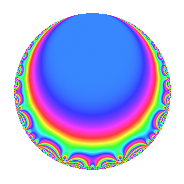# Properties

 Label 95.1.d.bLevel 95 Weight 1 Character orbit 95.d Self dual Yes Analytic conductor 0.047 Analytic rank 0 Dimension 2 Projective image $$D_{4}$$ CM disc. -95 Inner twists 4

# Related objects

## Newspace parameters

 Level: $$N$$ = $$95 = 5 \cdot 19$$ Weight: $$k$$ = $$1$$ Character orbit: $$[\chi]$$ = 95.d (of order $$2$$ and degree $$1$$)

## Newform invariants

 Self dual: Yes Analytic conductor: $$0.0474111762001$$ Analytic rank: $$0$$ Dimension: $$2$$ Coefficient field: $$\Q(\sqrt{2})$$ Coefficient ring: $$\Z[a_1, a_2]$$ Coefficient ring index: $$1$$ Projective image $$D_{4}$$ Projective field Galois closure of 4.2.475.1 Artin image size $$16$$ Artin image $D_8$ Artin field Galois closure of 8.2.4286875.1

## $q$-expansion

Coefficients of the $$q$$-expansion are expressed in terms of $$\beta = \sqrt{2}$$. We also show the integral $$q$$-expansion of the trace form.

 $$f(q)$$ $$=$$ $$q$$ $$-\beta q^{2}$$ $$+ \beta q^{3}$$ $$+ q^{4}$$ $$- q^{5}$$ $$-2 q^{6}$$ $$+ q^{9}$$ $$+O(q^{10})$$ $$q$$ $$-\beta q^{2}$$ $$+ \beta q^{3}$$ $$+ q^{4}$$ $$- q^{5}$$ $$-2 q^{6}$$ $$+ q^{9}$$ $$+ \beta q^{10}$$ $$+ \beta q^{12}$$ $$-\beta q^{13}$$ $$-\beta q^{15}$$ $$- q^{16}$$ $$-\beta q^{18}$$ $$- q^{19}$$ $$- q^{20}$$ $$+ q^{25}$$ $$+ 2 q^{26}$$ $$+ 2 q^{30}$$ $$+ \beta q^{32}$$ $$+ q^{36}$$ $$+ \beta q^{37}$$ $$+ \beta q^{38}$$ $$-2 q^{39}$$ $$- q^{45}$$ $$-\beta q^{48}$$ $$+ q^{49}$$ $$-\beta q^{50}$$ $$-\beta q^{52}$$ $$+ \beta q^{53}$$ $$-\beta q^{57}$$ $$-\beta q^{60}$$ $$- q^{64}$$ $$+ \beta q^{65}$$ $$-\beta q^{67}$$ $$-2 q^{74}$$ $$+ \beta q^{75}$$ $$- q^{76}$$ $$+ 2 \beta q^{78}$$ $$+ q^{80}$$ $$- q^{81}$$ $$+ \beta q^{90}$$ $$+ q^{95}$$ $$+ 2 q^{96}$$ $$+ \beta q^{97}$$ $$-\beta q^{98}$$ $$+O(q^{100})$$ $$\operatorname{Tr}(f)(q)$$ $$=$$ $$2q$$ $$\mathstrut +\mathstrut 2q^{4}$$ $$\mathstrut -\mathstrut 2q^{5}$$ $$\mathstrut -\mathstrut 4q^{6}$$ $$\mathstrut +\mathstrut 2q^{9}$$ $$\mathstrut +\mathstrut O(q^{10})$$ $$2q$$ $$\mathstrut +\mathstrut 2q^{4}$$ $$\mathstrut -\mathstrut 2q^{5}$$ $$\mathstrut -\mathstrut 4q^{6}$$ $$\mathstrut +\mathstrut 2q^{9}$$ $$\mathstrut -\mathstrut 2q^{16}$$ $$\mathstrut -\mathstrut 2q^{19}$$ $$\mathstrut -\mathstrut 2q^{20}$$ $$\mathstrut +\mathstrut 2q^{25}$$ $$\mathstrut +\mathstrut 4q^{26}$$ $$\mathstrut +\mathstrut 4q^{30}$$ $$\mathstrut +\mathstrut 2q^{36}$$ $$\mathstrut -\mathstrut 4q^{39}$$ $$\mathstrut -\mathstrut 2q^{45}$$ $$\mathstrut +\mathstrut 2q^{49}$$ $$\mathstrut -\mathstrut 2q^{64}$$ $$\mathstrut -\mathstrut 4q^{74}$$ $$\mathstrut -\mathstrut 2q^{76}$$ $$\mathstrut +\mathstrut 2q^{80}$$ $$\mathstrut -\mathstrut 2q^{81}$$ $$\mathstrut +\mathstrut 2q^{95}$$ $$\mathstrut +\mathstrut 4q^{96}$$ $$\mathstrut +\mathstrut O(q^{100})$$

## Character Values

We give the values of $$\chi$$ on generators for $$\left(\mathbb{Z}/95\mathbb{Z}\right)^\times$$.

 $$n$$ $$21$$ $$77$$ $$\chi(n)$$ $$-1$$ $$-1$$

## Embeddings

For each embedding $$\iota_m$$ of the coefficient field, the values $$\iota_m(a_n)$$ are shown below.

For more information on an embedded modular form you can click on its label.

Label $$\iota_m(\nu)$$ $$a_{2}$$ $$a_{3}$$ $$a_{4}$$ $$a_{5}$$ $$a_{6}$$ $$a_{7}$$ $$a_{8}$$ $$a_{9}$$ $$a_{10}$$
94.1
 1.41421 −1.41421
−1.41421 1.41421 1.00000 −1.00000 −2.00000 0 0 1.00000 1.41421
94.2 1.41421 −1.41421 1.00000 −1.00000 −2.00000 0 0 1.00000 −1.41421
 $$n$$: e.g. 2-40 or 990-1000 Significant digits: Format: Complex embeddings Normalized embeddings Satake parameters Satake angles

## Inner twists

Char. orbit Parity Mult. Self Twist Proved
1.a Even 1 trivial yes
95.d Odd 1 CM by $$\Q(\sqrt{-95})$$ yes
5.b Even 1 yes
19.b Odd 1 yes

## Hecke kernels

This newform can be constructed as the kernel of the linear operator $$T_{2}^{2}$$ $$\mathstrut -\mathstrut 2$$ acting on $$S_{1}^{\mathrm{new}}(95, [\chi])$$.